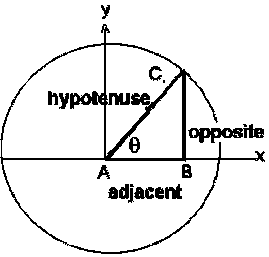# Trigonometry Calculator

The trigonometric functions have been defined using the unit circle.

 Sin Cos Tan Cot Sec Cosec
 Arc Sin Arc Cos Arc Tan Arc Cot Arc Sec Arc Cosec

#### Degrees

 Sin Cos Tan Cot Sec Cosec
 Arc Sin Arc Cos Arc Tan Arc Cot Arc Sec Arc Cosec

The sin(θ) is the vertical component , the cos(θ) is the horizontal coordinate of the arc endpoint.

where

Sin(q) = Opposite / Hypotenuse